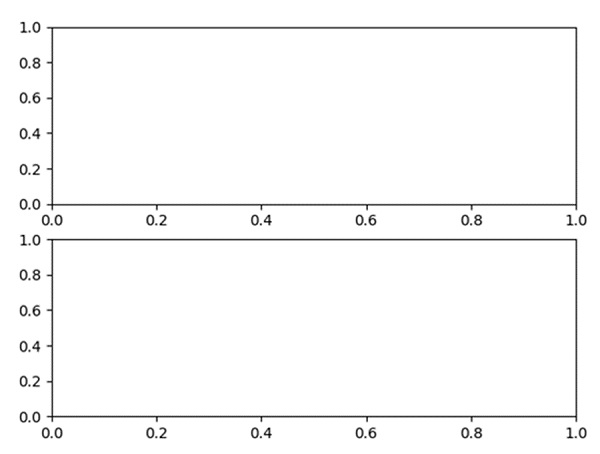# Row and column headers in Matplotlib's subplots

Using the subplot method, we can configure the number of rows and columns. nrows*nclos will create number positions to draw a diagram.

## Steps

• Number of rows = 2, Number of columns = 1, so total locations are: 2*1 = 2.

• Add a subplot to the current figure, nrow = 2, ncols = 1, index = 1.

• Add a subplot to the current figure, nrow = 2, ncols = 1, index = 2.

• Using plt.show(), we can show the figure.

## Example

from matplotlib import pyplot as plt

row_count = 2
col_count = 1
index1 = 1

# no. of subplots are: row*col, index is the position of figure.

plt.subplot(row_count, col_count, index1)

index2 = 2
plt.subplot(row_count, col_count, index2)

plt.show()

## Output